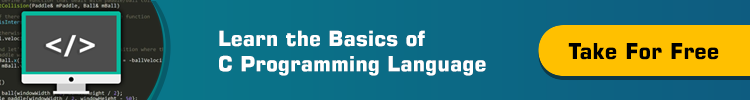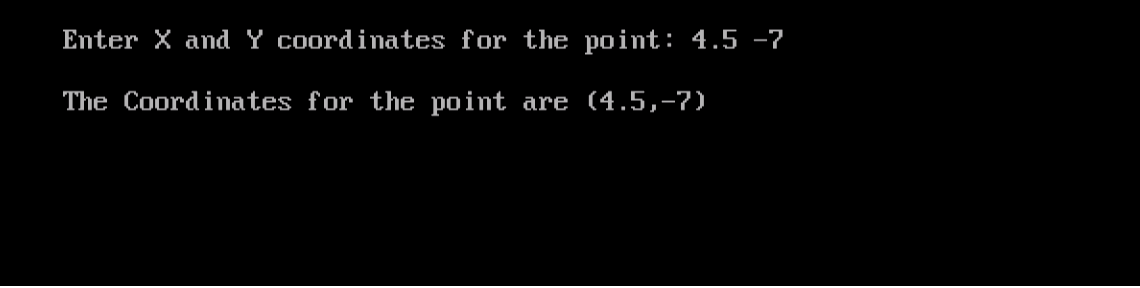# Learn the Concept of OOP in C++ Programming

0
3277What is OOP?
Welcome to the article you are all waiting for; Object-Oriented Programming.
Object-Oriented Programming is an approach that models real-life objects into programmable classes and objects.

– Sorry, that is not clear. Could you please make it simpler?

Okay, let’s say break it down: imagine you have a car, this car has some specifications: manufacturer, model, manufacturing year, number of doors, number of seats, engine, horse power, and color…

– Wait, I think we can group all these specifications into a structure, and we can call it car.

Good thinking from you. But, let me ask: isn’t your car capable of moving, accelerating, braking, and stopping?! How would you classify such actions?! Also, let me answer: these are called behavior. So, a car has both specs (we will refer to it as data or attributes), and behavior. Now, the question is: how could we represent, or simulate both the attributes and behavior of the car?

Object-Oriented Programming gives us the answer. Let me borrow Robert Lafore’s words from his great book “Object-Oriented Programming in C++”:
The fundamental idea behind object-oriented languages is to combine into a single unit both data (attributes) and the functions (behavior) that operate on that data. Such unit is called an object”.

Is it clear now?! Thank you.

Why OOP?
Besides providing the capability to model real-world objects into software-programmable objects, using the OOP approach has some benefits:

• Enhanced Security: the combination of both attributes and functions into one unit (object) is called encapsulation. Using encapsulation, we could restrict access to an object’s attributes to be only via its defined member functions. Needless to tell you that these functions are written by the one who has developed the object. So, access to object’s data is controlled. This prevents direct alteration of data, hence protects both, the confidentiality and the integrity of data.
• Creating User-Defined Data Types: so far, we have learned two ways to implement user-defined data types: Structures, and Enumerations. OOP provides the third. In OOP, you create a class, and give it a name, say for example car. Then, you could define as many objects of this class as you need. To better understand the idea, consider the following variable declaration:
```int counter;
```

An object’s relation to a class is the same as how the variable counter is related to its type int. So, an object is an instance of the class it belongs to.

• Inheritance: you wrote a class that implements about 90% of the requirements I need in a class I am about to write. So, why do I have to write a new one from scratch, while an existing one could almost do the job? The ability to modify and reuse other people’s classes saves time, increases productivity, and enriches the whole developing process.
• Code Reusability: by using classes developed by others, either directly, or after inheriting it and adding/modifying your own adjustments (based on your own needs). Again, this saves time by not having to re-invent the wheel, and enriches the developing the process.Writing our First Class
Fed up with theoretical talks?! Let’s then write our first OOP program.

Example
The following is our first contact with classes and objects. We will define a class called point. If you have studied geometry, you should know that a point in the plane is identified by two coordinates: x and y. let’s write a class for it.

```/*
Our First Class Program
Author: Eduonix
Date  : December 2015
*/
#include <iostream.h>
#include <conio.h>
class point
{
float x,y;
public:
void setx(float i)
{ x=i; }
void sety(float j)
{ y=j; }
float getx()
{ return x; }
float gety()
{ return y; }
};
void main()
{
clrscr();
point P;
float x1,y1;
cout << "\nEnter X and Y coordinates for the point: ";
cin >> x1 >> y1;
P.setx(x1);
P.sety(y1);
cout << "\nThe Coordinates for the point are (" << P.getx()
<< "," << P.gety() << ")" << endl;
getch();
}
```

Let’s see how this would behave:Now, a lot of things need explanation. Let’s explain them:

• Before using the class, it should be defined:
`class point`

Where point is the name of the class. This will be followed by the body of the class which is delimited by curly braces and terminated by a semi-colon.

• Between the braces, the class body is written:
• Member variables are declared. They should be private. If none specified, the default access to class members is private. Member variables and member functions that are marked private are only accessible from within the class.
`   float x,y;`

So, the two attributes (member variables) x and y are private.

• The public keyword starts a public section of the class definition:
`public:`

The four member functions setx(), sety(), getx(), and gety() are defined under this section. They provide the only way to read and modify values of the class data from outside the class. That is why they should be made public.

• In the main() function, an object (instance) P of the class point is defined:
`point P;`
• To set the values of the object P attributes (variables x and y in this case), the two member functions setx() and sety() are called:
```P.setx(x1);
P.sety(y1);
```

Again, the dot operator comes into the scene. But this time to refer to a member function in an object.

• To retrieve the values of the attributes of the object P, the two member functions getx(), and gety() are called:
```cout << "\nThe Coordinates for the point are (" << P.getx()
<< "," << P.gety() << ")" << endl;
```

Summary